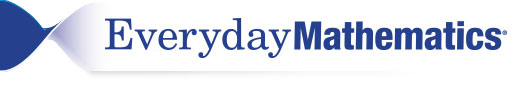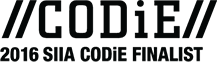# Program Materials

The Classroom Resource Package includes all the resources you need to teach the program. While you’ll never need to supplement the program, Everyday Mathematics 4 is flexible enough to allow you to add your own material to lessons and activities if you’d like.

Read more about our powerful teaching tools and student materials.## Digital Teacher and Student Resources

Everyday Mathematics 4 is the only research-­based program that can be implemented in a fully digital format, fully in print, or in a print-digital hybrid format—whichever fits your classroom. The digital resources in Everyday Mathematics 4 adapt to fit your needs and those of your students.

## Explore Everyday Mathematics

In Kindergarten, Everyday Mathematics focuses on procedures, concepts, and applications in two critical areas:

• Representing and comparing whole numbers, initially with sets of objects.
• Describing shapes and space.### Student Materials

In First Grade, Everyday Mathematics focuses on procedures, concepts, and applications in four critical areas:

• Understanding addition, subtraction, and strategies within 20.
• Understanding whole number relationships and place value, including grouping by tens and ones.
• Understanding linear measurement as iterating length units.
• Composing and decomposing geometric shapes and reasoning about the attributes of shapes.### Student Materials

In Second Grade, Everyday Mathematics focuses on procedures, concepts, and applications in four critical areas:

• Understanding of base-10 notation.
• Building fluency with addition and subtraction.
• Using standard units of measure.
• Describing and analyzing shapes.### Student Materials

In Third Grade, Everyday Mathematics focuses on procedures, concepts, and applications in four critical areas:

• Understanding of multiplication and division and strategies within 100.
• Understanding of fractions, especially unit fractions.
• Understanding of the structure of rectangular arrays and of area.
• Describing and analyzing two-dimensional shapes.### Student Materials

In Fourth Grade, Everyday Mathematics focuses on procedures, concepts, and applications in three critical areas:

• Understanding and fluency with multi-digit multiplication, and understanding of dividing to find quotients with multi-digit dividends.
• Understanding of fraction equivalence, addition and subtraction of fractions with like denominators, and multiplication of fractions by whole numbers.
• Understanding that geometric figures can be analyzed and classified based on their properties.### Student Materials

In Fifth Grade, Everyday Mathematics focuses on procedures, concepts, and applications in three critical areas:

• Developing addition/subtraction fluency with fractions, and understanding of multiplication/division of fractions in limited cases.
• Developing fluency with decimal operations, extending division to 2-digit divisors, integrating decimals into the place-value system, and understanding operations with decimals to hundredths.
• Developing an understanding of volume.### Student Materials

In Sixth Grade, Everyday Mathematics focuses on procedures, concepts, and applications in four critical areas:

• Connection ratio and rate to whole number multiplication and division.
• Completing understanding of division of fractions and expanding to a system of rational numbers including negative numbers.
• Writing, interpreting, and using expressions and equations.
• Developing understanding of statistical thinking.